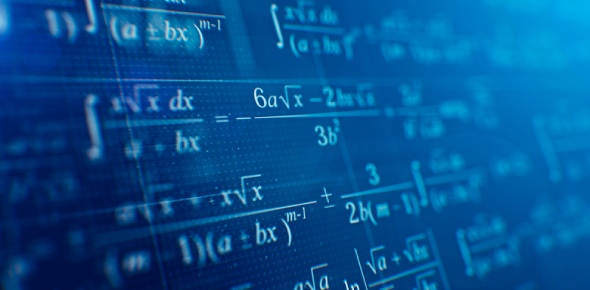# Inverse Function Mathematics Quiz

10 Preguntas | Total Attempts: 196SettingsIf you are a student, this quiz is for you, especially you are dealing with the inverse function in mathematics. Math is actually very interesting and easy with a lot of practice. Try it out and see how much you know about it.

• 1.
F(x)=2x+4 and g(x)=(1/2)x-4 are inverses
• A.

True

• B.

False

• 2.
A vertical translation of f(x)=e^x changes its asymptote.
• A.

Always True

• B.

Sometimes True

• C.

Never True

• 3.
The traded-in value of Cindy's car is \$4500 and decreases by about 40% per year. Which choice represents the trade-in value as a function of time?
• A.

F(t)=4500(0.4)^t

• B.

F(t)=4500(0.6)^t

• C.

F(t)=4500(1.4)^t

• 4.
Electric power for the Cassini spacecraft is provided by the decay of plutonium-238 contained inside its generators. The power output of the generators in watts is modeled by P(t)=870e^(-t/127), where t is the number of years since the manufacture date. Describe how the model is transformed from its parent function. Suppose the instruments require at least 600 watts to function. Use the model to predict the number of years that the instruments on Cassini will function properly.
• A.

Ry,Sx:127;47

• B.

Ry,Sy:127;23

• C.

Rx,Tx:127;79

• 5.
Which list shows the functions in order form the most compressed horizontally from f(x)=log x to the most stretched?
• A.

F(x)= log(x-10), f(x)= log(10x), f(x)=log(x/10)

• B.

F(x)= log(x/10), f(x)= log(x-10), f(x)=log(10x)

• C.

F(x)= log(10x), f(x)= log(x-10), f(x)=log(x/10)

• 6.
Find the inverse function of y = 9x.
• A.

Y=9/x

• B.

Y=9x

• C.

Y=x/9

• D.

Y=3/x

• 7.
A spaceship takes off.  Its speed at x minutes after launch is given by the function, y=log(5x/4)+35.  Find its inverse
• A.

X=(10^y)+35/5

• B.

X=log(4*10^(y-35))

• C.

X=log(5y/4)+35

• D.

X=(4*10^(y-35))/5

• 8.
Find the equation of the function (in blue) and its inverse (in red) shown in the graph.
• A.

Y = (x + 2)^2,

• B.

, y=13x^2

• C.

Y=(x+5)^2, y=5/2x

• D.

Y=(x/32)^2 y=32/2x

• 9.
The population of a new species of frog, Litoria Algebrus was found with a population of 457 frogs.  After some research, scientists found that the population of the frog had an annual increase of 5.7%, starting in 1998.  Find the inverse of the function, and the year that the population reaches 1172
• A.

Y=log(x/457)/log(1.057), 1999

• B.

Y=log(x)/log(1.057), 2020

• C.

Y=log(x/457)/log(1.057), 2015

• D.

Y=log(x/457)/log(1.057), 2004

• 10.
Find the inverse of (x^4-15x^2-10x+24)/(x^2+x-12)
• A.
• B.
• C.
• D.
Related TopicsBack to top